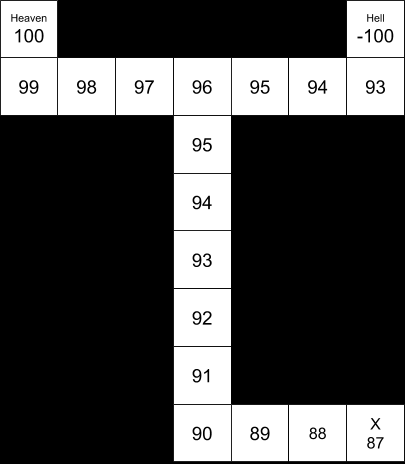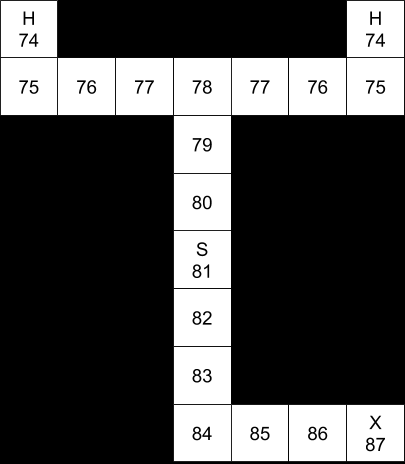# Heaven, Hell, and Markov Decision Processes

I’ve been thumbing through Sebastian’s magnum opus, Probabilistic Robotics. The book is now 13 years old, but it remains a great resource for roboticists. Kind of funny to think that, when Sebastian wrote this, he hadn’t even started to work on self-driving cars yet!

The chapter on Markov decision processes (MDPs) covers how to make robotic planning decisions under uncertainty. One of the key assumptions of MDPs is that the agent (robot) can observe its environment perfectly. This turns out to be an unrealistic assumption, which leads to further types of planning algorithms, principally partially observable Markov decision processes (POMDPs).

Nonetheless, ordinary Markov decision processes are a helpful place to start when thinking about motion planning.

I love the exercises at the end of each chapter of Probabilistic Robotics. There is a fun one called “Heaven or Hell” at the end of the MDP chapter. This is actually a variation on a toy problem long-used in the field of motion planning.

#### Heaven or Hell?

“In this exercise, you are asked to extend dynamic programming to an environment with a single hidden state variable. The environment is a maze with a designated start marked “S”, and two possible goal states, both marked “H”.

“What the agent does not know is which of the two goal states provides a positive reward. One will give +100, whereas the other will give -100. There is a .5 probability that either of those situations is true. The cost of moving is -1; the agent can only move into the four directions north, south, east, and west. Once a state labeled “H” has been reached, the play is over.”

So far, so good.

“(a) Implement a value iteration algorithm for this scenario. Have your implementation compute the value of the starting state. What is the optimal policy?”

The optimal policy here depends on whether we assume the agent must move. If the agent is allowed to remain stationary, then the value of the starting state is 0, because the optimal policy is to stay put.

Calculating the expected reward from reaching state “H” is straightforward. The expected reward is 0, because there’s a 50% chance of a +100 reward, but also a 50% chance of a -100 reward.

0.5 * (+100) + 0.5 * (-100) = 50 + (-50) = 0

Once we establish that, the optimal policy is intuitive. There is no positive reward for reaching any state, but there is a cost to moving to any state. Don’t incur a cost if there’s no possible reward.

The optimal policy changes, however, if the rules state that we must move. In that case, we want to end the game as quickly as possible.

Under this set of rules, the value function decreases as we approach either “H”. The intuition is that the game has no benefits, only costs, so we want to end the game as quickly as possible. From a policy perspective, we want to follow the gradient toward higher values, so if we start at “S”, we wind up trending toward “H”.

“(b) Modify your value algorithm to accommodate a probabilistic motion model: with 0.9 chance the agent moves as desired; with 0.1 chance it will select any of the other three directions at random. Run your value iteration algorithm again, and compute both the value of the starting state, and the optimal policy.”

Once again, the optimal policy depends on whether we can remain stationary. If we can remain stationary, then the value of all cells is 0, and the optimal policy is to stay put. The uncertainty in motion that has just been introduced does not affect the policy, because there’s still no reward for moving anywhere.

If, however, we are required to move, calculating the policy becomes more complex. At this point we really need a computer to calculate the value function, because we have to iterate over all the cells on the map until values converge. For each cell, we have to look at each action and sum the 90% probability that the action will execute properly, and the 10% probability that the action will misfire randomly. Then we pick the highest-value action. Once we do this for every cell, we repeat the cycle over all the cells again, and we keep doing this until the values stabilize.

The first pass in the iteration sets all cells to 0. Depending on which direction we iterate from, the next step might look like this:

Nonetheless, even without a computer, it seems pretty clear that the optimal policy is still for our agent to stay put in the start cell. Without any information about which “H” is heaven and which is hell, there’s no ultimate reward for going anywhere.

“(c) Now suppose the location labeled X contains a sign that informs the agent of the correct assignment of rewards to the two states labeled “H”. How does this affect optimal policy?”

Without computing the policy, it seems likely that the optimal policy will involve going to the sign, identifying heaven and hell on the map, and then proceeding to heaven.

This policy seems qualitatively clear because of the relatively high payoff for reaching heaven (+100), the relatively low cost of motion (-1), the relatively high probability of the motion executing accurately (0.9), and the relatively small size of the map (distance from S to X to H = 19).

It’s easy to imagine tweaking these parameters such that it’s no longer so obvious that it makes sense to go find the sign. With different parameters, it might still make sense to stay put at S.

“(d) How can you modify your value iteration algorithm to find the optimal policy? Be concise. State any modifications to the space over which the value function is defined.”

Basically, we need to figure out the value of reaching the sign. There are essentially two value functions: the value function when we cannot observe the state, and the value function when we can.

Another way to put this is that going to the sign is like taking a measurement with a sensor. We have prior beliefs about the state of the world before we reach the sign, and then posterior beliefs once we get the information from the sign. Once we transition from prior to posterior beliefs, we will need to recalculate our value function.

An important point here is that this game assumes the sign is 100% certain, which makes the model fully observable. That’s not the case with normal sensors, which is why real robots have to deal with partially observable Markov decision processes (POMDPs).

“(e) Implement your modification, and compute both the value of the starting state and the optimal policy.”

Again, we’d need to write code to actually implement this, but the general idea is to have two value functions. The value of X will be dependent on the posterior value function (the value function that we can calculate once we know which is heaven and which is hell). Then we use that value of X to calculate our prior distribution.

For example, here are the value functions, assuming perfect motion:The posterior value function, after reading the sign at “X”.The prior value function, before reading the sign at “X”.